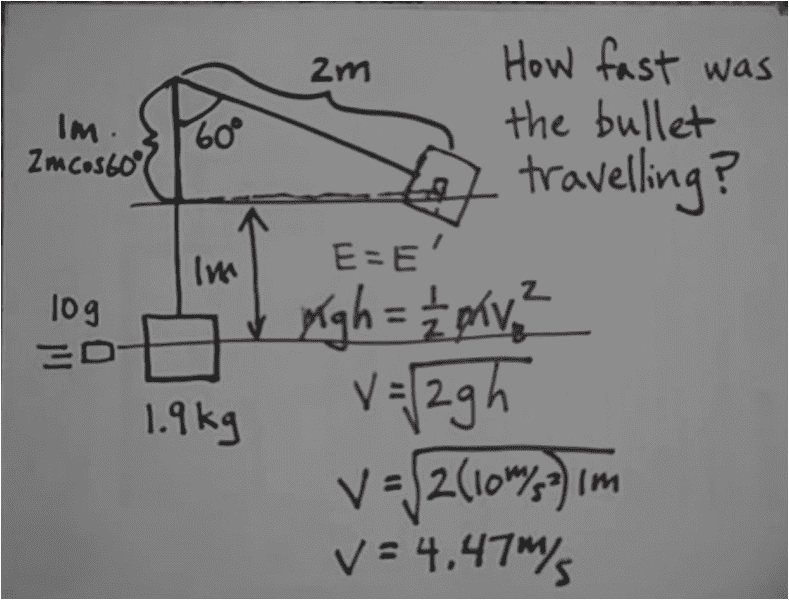# A Curious question About Ballistic Pendulum Solution

I've watched a video about ballistic pendulum problems.
The illustration is :I wonder why the final energy is just a gravity potential energy.
I think there is also a rotational kinetic energy (since it will rotate and oscillate)
Is my thought wrong?

Bystander
Homework Helper
Gold Member
also a rotational kinetic energy
What's the rotational velocity at the "peak" of the pendulum displacement?

What's the rotational velocity at the "peak" of the pendulum displacement?
I'm not quite sure. But, I think it will be v/r
Am I right ?

Bystander
Homework Helper
Gold Member
In your sketch, where the maximum displacement from the vertical is indicated to be sixty degrees, how fast is the pendulum moving?

•terryds
In your sketch, where the maximum displacement from the vertical is indicated to be sixty degrees, how fast is the pendulum moving?
Do you mean the velocity ?
I think it's 0 m/s since it has reached the amplitude.